# Density Chart with Multiple Groups

This post shows how to compare the distribution of several variables with density charts using the kdeplot() function of seaborn library. Here are a few examples with descriptions.

## Density Chart with Multiple Groups

A multi density chart allows to compare the distribution of several groups. Unfortunatelly, this type of charts tend to get cluttered: groups overlap each other and the figure gets unreadable. An easy workaround is to use transparency. However, it won’t solve the issue completely and it is often better to consider other options suggested further in this post.

``````# libraries
import seaborn as sns
import matplotlib.pyplot as plt
from plotnine.data import diamonds # dataset

# Set figure size for the notebook
plt.rcParams["figure.figsize"]=12,8

# set seaborn whitegrid theme
sns.set(style="whitegrid")

# Without transparency
sns.kdeplot(data=diamonds, x="price", hue="cut", cut=0, fill=True, common_norm=False, alpha=1)
plt.show()``````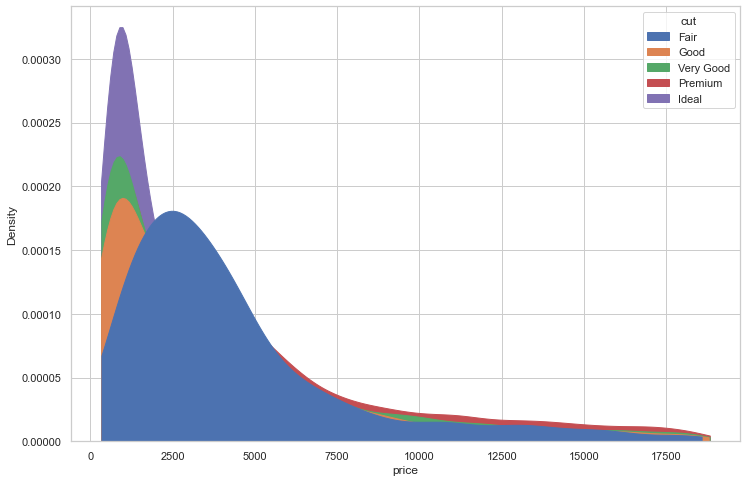Note you can easily produce pretty much the same figure with some more transparency in order to see all groups

``````# With transparency
sns.kdeplot(data=diamonds, x="price", hue="cut", fill=True, common_norm=False, alpha=0.4)
plt.show()``````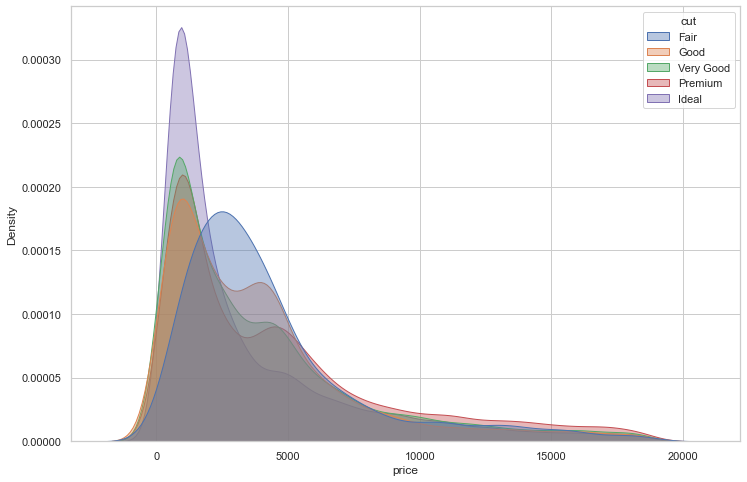Here is an example with another dataset where it works much better. In this dataset, groups have very distinct distribution, and it is easy to spot them even if on the same chart. Note that it is recommended to add group name next to their distribution instead of having a legend beside the chart.

``````# libraries
import seaborn as sns
import matplotlib.pyplot as plt
import pandas as pd

# set seaborn whitegrid theme
sns.set(style="whitegrid")

# load dataset from github and convert it to a long format
data = pd.melt(data, var_name='text', value_name='value')

# take only "Almost No Chance", "About Even", "Probable", "Almost Certainly"
data = data.loc[data.text.isin(["Almost No Chance","About Even","Probable","Almost Certainly"])]

# density plot
p = sns.kdeplot(data=data, x="value", hue="text", fill=True, common_norm=False, alpha=0.6, palette="viridis", legend=False)
# control x limit
plt.xlim(0, 100)

# dataframe for annotations
annot = pd.DataFrame({
'x': [5, 53, 65, 79],
'y': [0.15, 0.4, 0.06, 0.1],
'text': ["Almost No Chance", "About Even", "Probable", "Almost Certainly"]
})

# add annotations one by one with a loop
for point in range(0,len(annot)):
p.text(annot.x[point], annot.y[point], annot.text[point], horizontalalignment='left', size='large')

plt.xlabel("Assigned Probability (%)")
plt.ylabel("")

# show the graph
plt.show()``````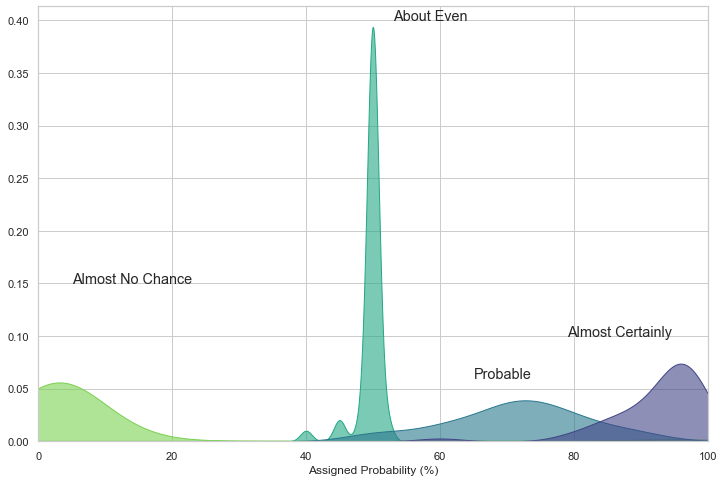## Small Multiples

By using small multiple, it gets easy to read the distribution of each group. It is still possible to compare groups since they share the same X axis boundaries. The faceting is made using the awesome `FacetGrid()` utility of seaborn.

``````# libraries
import seaborn as sns
import matplotlib.pyplot as plt
from plotnine.data import diamonds  # dataset

# set seaborn whitegrid theme
sns.set(style="whitegrid")

# using small multiple
# create a grid
g = sns.FacetGrid(diamonds, col='cut', hue='cut', col_wrap=3)

# draw density plots
g = g.map(sns.kdeplot,"price", cut=0, fill=True, common_norm=False, alpha=1, legend=False)

# control the title of each facet
g = g.set_titles("{col_name}")

# show the graph
plt.show()``````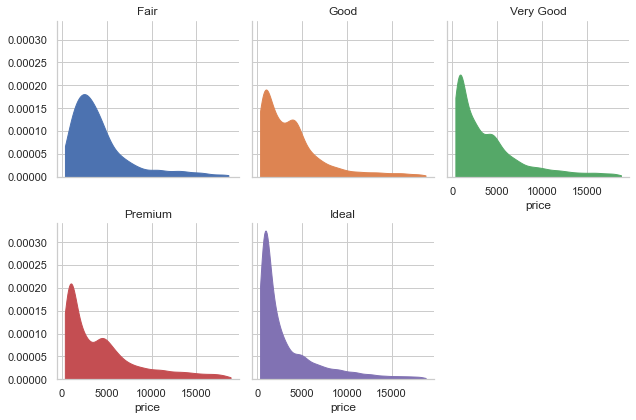## Stacked Density Chart

Another solution is to stack the groups by passing "fill" to the `multiple` argument of the function. This allows to see which group is the most frequent for a given value, but it makes hard to understand the distribution of a group that is not on the bottom of the chart.

You can visit data to viz for a complete explanation on this matter.

``````# libraries
import seaborn as sns
import matplotlib.pyplot as plt
from plotnine.data import diamonds # dataset

# set seaborn whitegrid theme
sns.set(style="whitegrid")

# stacked density plot
sns.kdeplot(data=diamonds, x="price", hue="cut", common_norm=False, multiple="fill", alpha=1)

# show the graph
plt.show()``````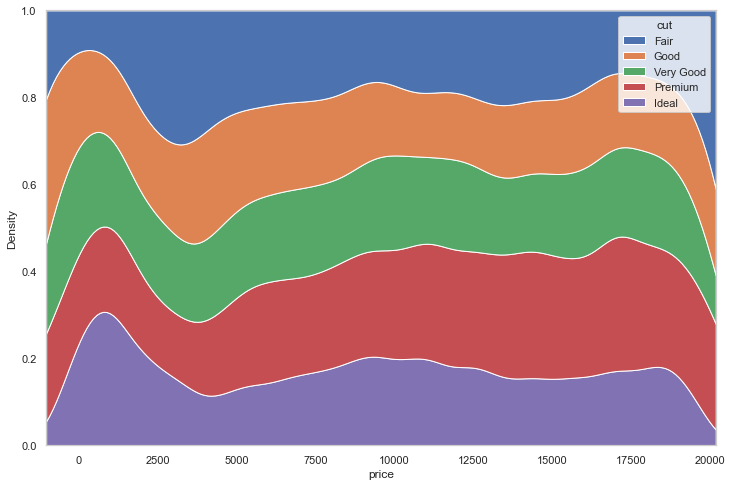## Contact & Edit

👋 This document is a work by Yan Holtz. Any feedback is highly encouraged. You can fill an issue on Github, drop me a message onTwitter, or send an email pasting `yan.holtz.data` with `gmail.com`.